MEDIUM
DATA STRUCTURES AND ALGORITHMS

# How to Solve Top K Frequent Elements (with Solutions in Python, Java & JavaScript)

Written ByTom WagnerKenny Polyak

## Introduction to the Top K Frequent Elements Problem

The Top K Frequent Elements problem involves finding the K most frequent elements in a given dataset. It is a useful problem for data analysis and can be used to identify trends in data. Solutions to this problem include advanced techniques such as implementing a heap or quickselect, making it a useful problem for testing a broad range of sorting approaches and their complexity tradeoffs.

## Examples of the Top K Frequent Elements Problem

Given an integer array `nums` and an integer `k`, return the `k` most frequent elements. You may return the answer in any order.

### Example 1

Input: nums = [1,1,1,2,2,3], k = 2 Output: [1,2] or [2,1]

### Example 2

Input: nums = , k = 1 Output: 

Constraints

• 1 <= nums.length <= 105
• -104 <= nums[i] <= 104

## How to Solve Top K Frequent Elements: 3 Approaches

This problem falls into a category sometimes referred to as a k-selection problem. Typically, we are given a collection of elements that we are asked to manipulate in some way (sort, group, process, etc), and then our final output is modified by a parameter `k`. Due to the nature of this kind of problem, we can imagine solving it with `k` as a constant - "return the most frequent element" or "return the two most frequent elements" - and then consider how to solve it with `k` as a variable. (In some cases, an interviewer might even offer the `k` parameter as a follow-up to a simpler problem). We can arrive at brute force approach by simplifying the problem, which will then help us explore optimizations using the `k` parameter.

### 1. Brute Force

Let's start by thinking about how we would solve this problem without a computer. After all, the ask here is very practical and probably something we've had to do in our material human lives. Let's imagine we're going to bring some fruit to a potluck; we look in our fruit basket at home and find some apples, oranges, and bananas. Right before we head out, our friend calls and says to only bring two fruit varieties, since someone else is also bringing fruit, but we should still try to bring as much fruit as possible. This high pressure task doesn't phase us, since we are expert problem solvers!

What are the simplest steps we can take to figure out what we need? Firstly, we should get organized: currently the basket is arranged randomly, so let's batch the fruit by variety and count the number of units for each. Secondly, since we want to bring the most fruit, let's sort the varieties based on count in descending order. Finally, we identify the first `k` varieties (in this case, our friend asked for the top two) from the sorted list and bring only these fruits.

Thinking algorithmically, we can see that the efficiency of our code is limited by the sort. Building the counts hash map is O(n) time, and sorting the subsequent list is O(n log n).

#### Top K Frequent Elements Python and JavaScript Solutions - Sorting

``````class Solution:
def top_k_frequent(self, nums: List[int], k: int) -> List[int]:
# 1: Count the frequency of each element in the input list
counts = {}
for num in nums:
if num not in counts:
counts[num] = 1
else:
counts[num] += 1

# 2: Sort in descending order based on the counts
counts_list = sorted(counts.items(), key=lambda x:x, reverse=True)
# 3: Slice the first k elements to build the result list
sorted_counts = dict(counts_list[:k])

return [num for num in sorted_counts]``````

#### Time/Space Complexity

• Time Complexity: `O(n log(n))`, where `n` is the number of elements in nums. We use O(n) time to build the counts hash, and O(n log n) to sort the hash.
• Space Complexity: `O(n)`, for the counts hash map with `n` elements.

### 2. Heap

The brute force approach is a relatively straightforward sorting solution - the interviewer will likely be interested in seeing how you identify optimizations for this sort using the `k` parameter. Usually, you're not expected to propose alternative sorting algorithms - in fact, we can typically just rely on the native sort algorithms in our language of choice, and assume an O(n log n) time complexity for sorting `n` elements.

Instead, one possible optimization for sorting is to identify where we are doing unnecessary work; namely, if we are sorting too many elements on each sort. This kind of k-selection problem is a prime candidate for this line of questioning. Since we are asked to return only `k` elements from a list of `n` elements, can we avoid sorting the entire list all at once? With the use of a priority queue, we can do just that.

As a quick recap, a priority queue or a heap is a sorted data structure resembling a binary tree that is backed by an array; practically speaking, the tree is not exposed to the caller, and instead we can imagine it's a kind of self-sorting array. Typically, heaps are implemented to store elements in either descending order - a max-heap - or in ascending order - a min-heap, and when elements are added or removed, the heap maintains its sorted order. We can usually assume access to a black box heap class in an interview setting (so long as the task itself is not to build a heap), and just discuss the features and api offered by this data structure. It's important to realize that, although the implementation can be complex, a heap is a very simple tool in practice. It's basically just a list that is sorted every time an element is added or removed, which is why we can often use a basic list or array to represent it.

• peek - O(1): get the largest/smallest element in the collection
• pop - O(1): remove the largest/smallest element in the collection
• insert - O(log n): insert an element

So how does the heap help us with optimization? Recall that in the brute force approach, we sorted the entire count frequency list - size `n` - so we could identify the `k` most frequent elements, costing us O(n log n) time. But since we are only interested in the top `k` elements, we should only need to sort at most `k` elements at any given time, since that's all we need to end up with. Even sorting multiple times, but on a smaller set, will improve our time complexity given how expensive sorting is in the worst case: so long as `k` is smaller than `n`, sorting on a limited set will afford us an optimized O(n log k) time complexity. The vital piece of our algorithm is in maintaining the heap size of `k`.

Using a min heap to sort for some maximum values is pretty clever. The trick is that the heap will be performing a sort `n` times, but only ever on `k` elements at once. Iterating on the input list, we add elements to the heap one-by-one. Once the heap size is `k` + 1, we use the min heap to identify the element with the least frequency and remove that element (this element is necessarily not a member of the top `k` most frequent). We continue adding elements until we run out, at which point the remaining elements in the heap are the final result.

#### Top K Frequent Elements Python and JavaScript Solutions - Heap

``````import heapq
from collections import Counter
class Solution:
def topKFrequent(self, nums: List[int], k: int) -> List[int]:
# 1: Use a Counter collection for frequency counts - this produces the same outcome as our verbose implementation in the brute force approach.
counts = Counter(nums)
# 2: Initialize a min heap
min_heap = []

for element, freq in counts.items():
# 3: Manage the heap
# Add each element to the heap
heappush(min_heap, (freq, element))
# If the size of the heap is larger than k, we pop the element at the top.
if len(min_heap) > k:
heappop(min_heap)

# 4: What remains in the heap are the top k most frequent
return [num for (count, num) in min_heap]``````

#### Time/Space Complexity

• Time Complexity: `O(n log(k))`, where `n` is the number of elements in nums.
• Space Complexity: `O(n + k)`, for the counts hash map with `n` elements and the heap of size `k`

### 3. Quickselect

There exists yet another classic approach to k-selection problems - the quickselect algorithm. But before diving into this approach, it may be worth discussing it with your interviewer. For one, although the average time complexity is `O(n)`, the worst-case is `O(n²)` (though the probability is negligible and it depends on the input size, relative order, and values). Secondly, implementing quickselect can be more time consuming than simply referencing a heap library, so it may be in your best interest to avoid this approach, as long as your interviewer is not specifically interested in a quickselect solution; in fact, they may even be expecting heap. But in the pursuit of knowledge, let's take a look at quickselect.

A close relative to quicksort, quickselect works by repeatedly partitioning a list on a randomly selected pivot point, moving elements "less than" the pivot to the left side of the partition, and elements "greater than" to its right, until a target index is found. How does this help us find the k'th most frequent element? We can't really answer without a refresher on quicksort.

Quicksort is a divide and conquer algorithm that follows a fairly simple playbook:

1. Pivot selection: an element is picked from a list, often at random, though sometimes the leftmost or rightmost.
2. Parition: iterate through the list and move all elements smaller than the pivot element to its left, larger elements to its right, and equals wherever. Many different implementations exist for partitioning, all with tradeoffs.
3. Recurse: repeat these steps on both partitions, until the base case is reached, which is a single element (sorted by default).

A few things that could be worth mentioning in an interview:

• First, this sort is not stable, meaning if there are duplicate keys the algorithm does not guarantee their original order is preserved.
• Second, as the worst-case time complexity was mentioned above, there is a way to mitigate some of this risk by using random partition selection. The main reason is that the time complexity for this algorithm suffers greatly for nearly sorted lists - so, the approach we'll use below, called the Lomuto Partition Scheme, is to select a random pivot and swap it with the last position of the block, then perform the partitioning as usual. We won't go into depth into the time complexity analysis, but this method offers quicksort a favorable `O(n * log(n))` average runtime. Yet another partitioning approach is Hoare's Partition, which you can learn more about here, but this is trickier to implement in an interview.
• Finally, we should also assume none or very few duplicates, as a duplicate heavy search space is unavoidably `O(n²)`.

Let's move back to the big picture of quickselect - the main difference with quicksort is that we're not actually trying to sort the whole list. Instead, since we're searching for a specific index in the list, we only need to recurse (perform quicksort) on the partition that contains our target index - this is what affords us an average `O(n)` runtime. Quickselect takes advantage of a convenient mechanic from quicksort: after we've partitioned around a given pivot, that pivot is guaranteed to be in its correct place in the list. Therefore, once the pivot index is equal to our target index, we know that all elements to its right are larger (in this case, more frequent), with no need to recursively sort the partition.

#### Quickselect Example

The following images show our implementation of the quickselect partitioning algorithm in action.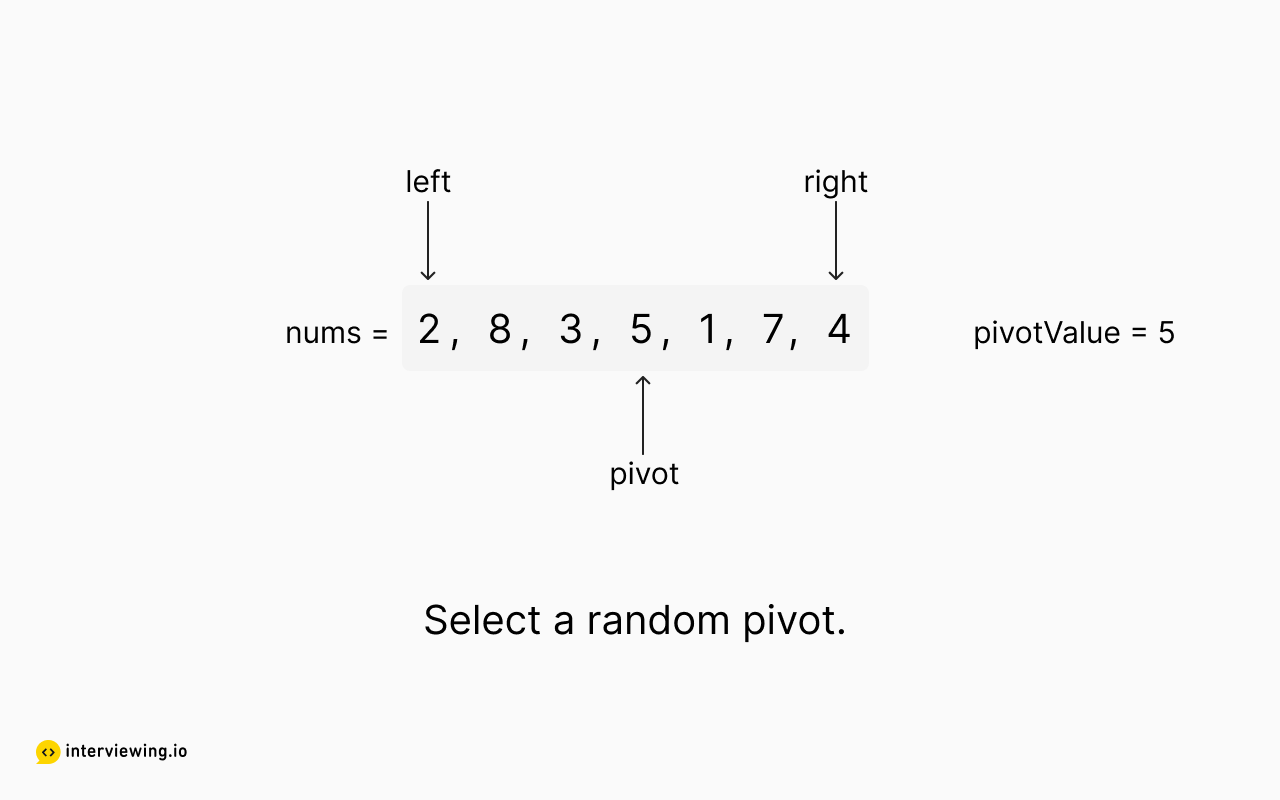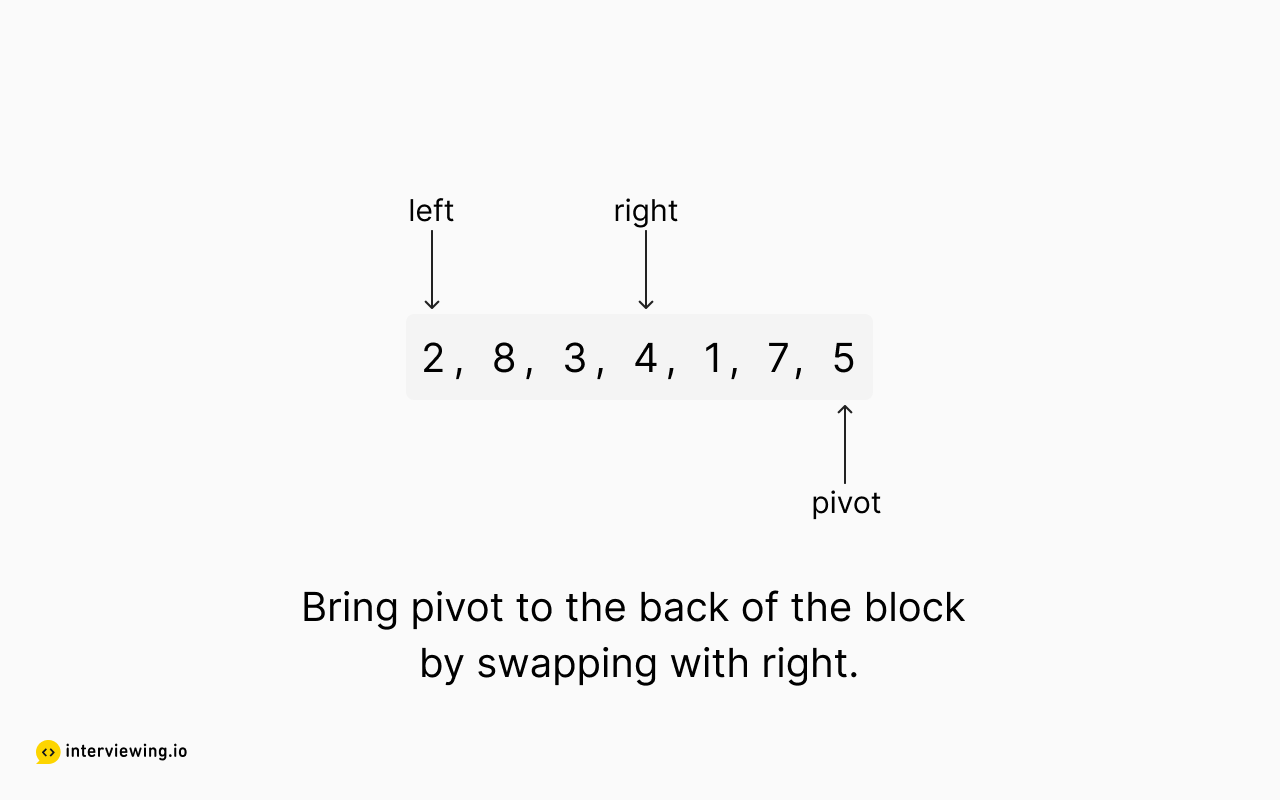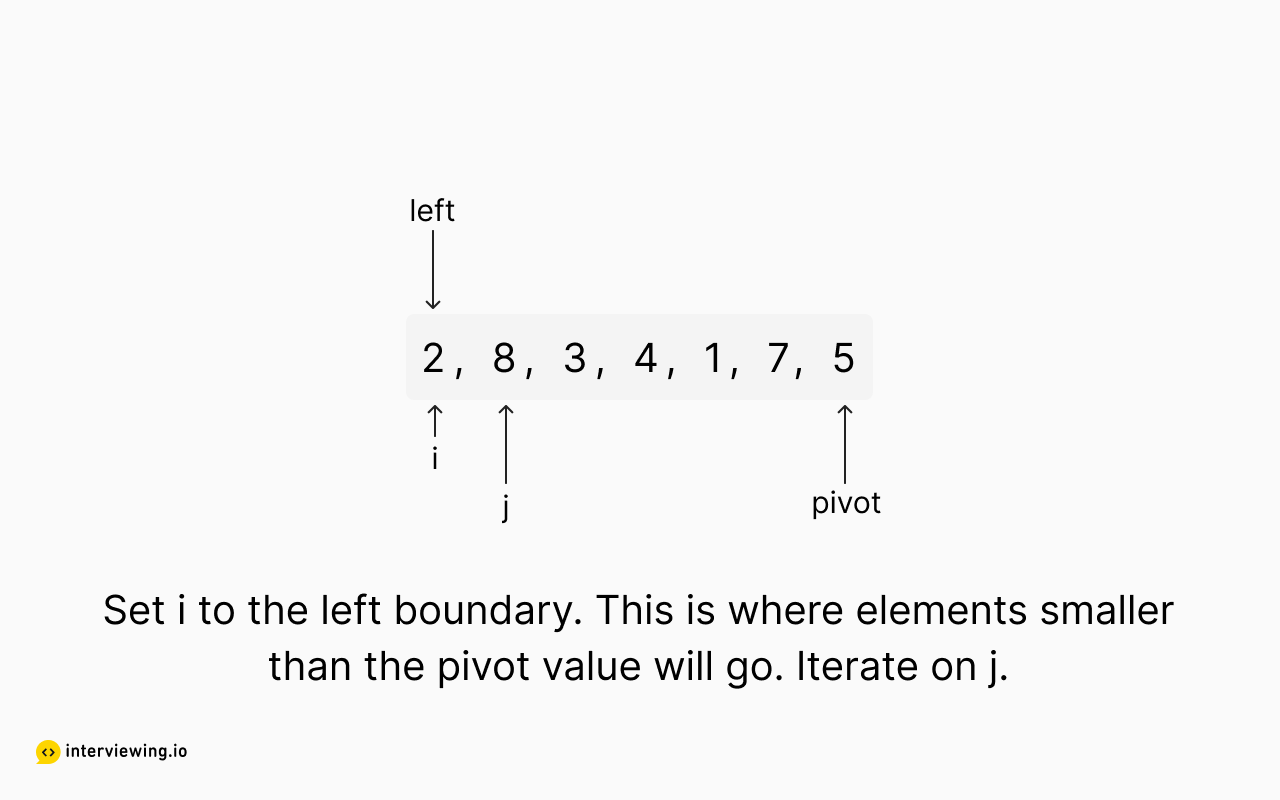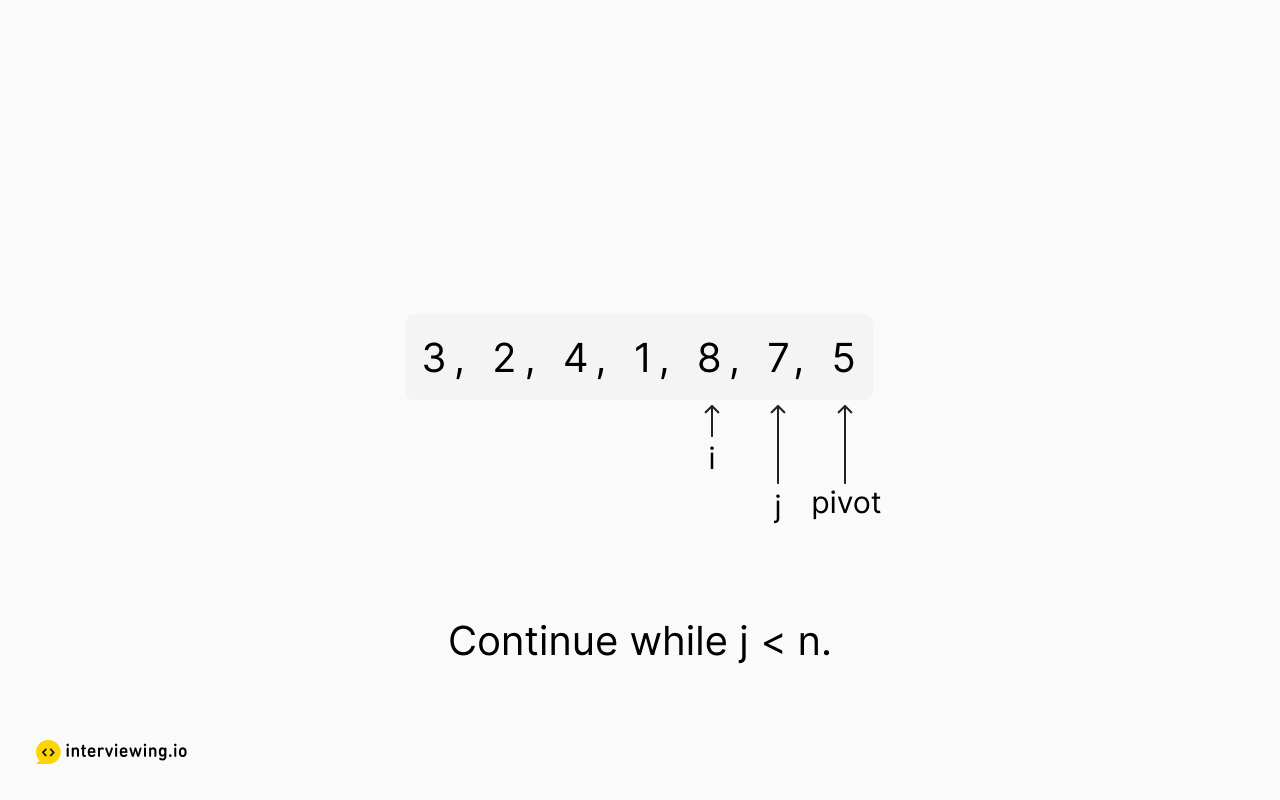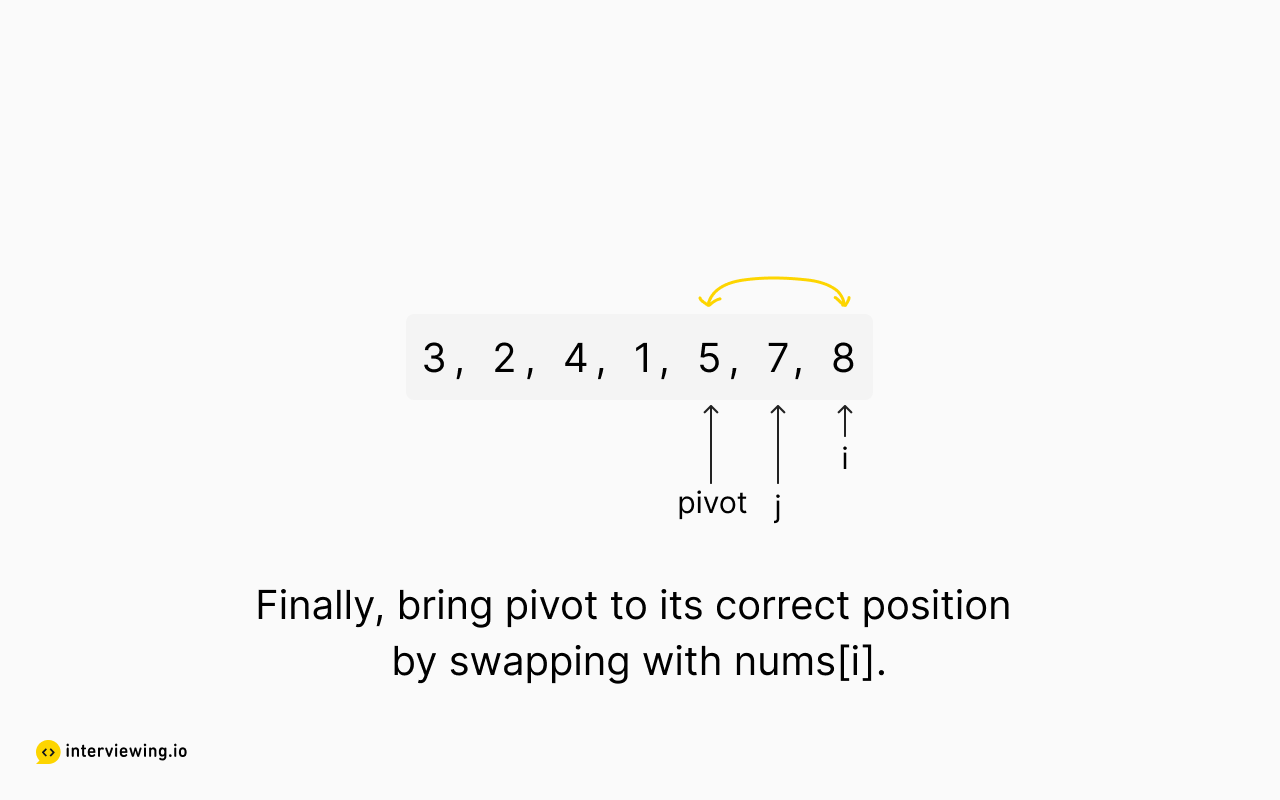#### Top K Frequent Elements Python and JavaScript Solutions

``````class Solution:
def partition(self, left: int, right: int, counts: list[tuple[int, int]]):
# get random pivot index
pivot_index = random.randint(left, right)
pivot_value = counts[pivot_index]
# move pivot element to the end
counts[pivot_index], counts[right] = counts[right], counts[pivot_index]
# when we find an element less than pivot_value, move it left of pivot_index and increment the swap position
i = left
for j in range(left, right):
if counts[j] < pivot_value:
counts[i], counts[j] = counts[j], counts[i]
i += 1
# move pivot to its final place
counts[right], counts[i] = counts[i], counts[right]
return i

def quickselect(self, left: int, right: int, k_target_index: int, counts: list[tuple[int, int]]):
# base case: if there is only one element in the partition, it's already sorted
if left == right:
return
# find the pivot's correct position
pivot_index = self.partition(left, right, counts)
# if the pivot index is equal to our target, we're done
if k_target_index == pivot_index:
return
elif k_target_index < pivot_index:
self.quickselect(left, pivot_index - 1, k_target_index, counts)
else:
self.quickselect(pivot_index + 1, right, k_target_index, counts)

def topKFrequent(self, nums: List[int], k: int) -> List[int]:
# get frequency counts
counts_dict = Counter(nums)
counts = list(counts_dict.items())

n = len(counts)

# since we are sorting in ASC order, the kth most frequent element is the (n - k)th element
self.quickselect(0, n - 1, n - k, counts)
return [count for count in counts[n - k:]]``````

#### Time/Space Complexity

• Time Complexity: `O(n)` in average, `O(n²)` in worst-case (dependent on the input shape).
• Space Complexity: `O(n)` for the counts hash map.

## Practice the Top K Frequent Elements Problem With Our AI Interviewer

Start AI Interview